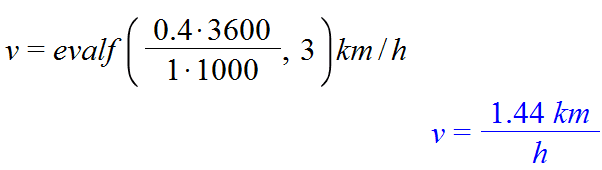# Confusion regarding significant figures in answers

## Homework Statement

I'm working through some exercises in which I'm supposed to convert various units of speed from SI units to other units
Example: 0.4m/s into km/h

## Homework Equations## The Attempt at a Solution

Having checked, I know my answer to be (to 3 significant figures) correct. My confusion arises regarding significant figures. Wouldn't 0.4 be 1 significant figure, which, if I remember and understand correctly, influences how the answer must be expressed, which in turn would leave me with a answer value of 1.

QuantumQuest
Gold Member
Doesn't the exercise state explicitly how many significant figures are needed for the result?

## Homework Statement

I'm working through some exercises in which I'm supposed to convert various units of speed from SI units to other units
Example: 0.4m/s into km/h

## Homework Equations

View attachment 112152

## The Attempt at a Solution

Having checked, I know my answer to be (to 3 significant figures) correct. My confusion arises regarding significant figures. Wouldn't 0.4 be 1 significant figure, which, if I remember and understand correctly, influences how the answer must be expressed, which in turn would leave me with a answer value of 1.

It's okay. I worked out where I was going wrong.

It's okay. I worked out where I was going wrong.
did you get the answer? when you are multiplying 2(or more) terms, the answer should contain as much as significant figures which is equivalent to that of the least number of significant figures containing term in the question.

did you get the answer? when you are multiplying 2(or more) terms, the answer should contain as much as significant figures which is equivalent to that of the least number of significant figures containing term in the question.
oops sorry, just now i have seen that it's marked solved.ignore my useless rambling then :p